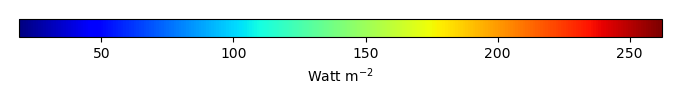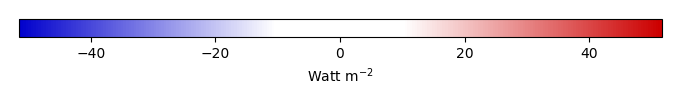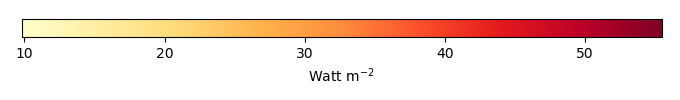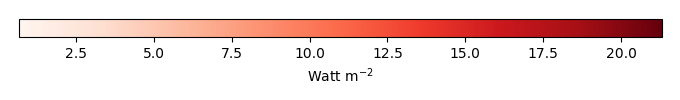# Mean State

Period Mean (original grids) [Watt m-2]
Model Period Mean (intersection) [Watt m-2]
Model Period Mean (complement) [Watt m-2]
Benchmark Period Mean (intersection) [Watt m-2]
Benchmark Period Mean (complement) [Watt m-2]
Bias [Watt m-2]
RMSE [Watt m-2]
Phase Shift [months]
Bias Score 
RMSE Score 
Seasonal Cycle Score 
Spatial Distribution Score 
Interannual Variability Score 
Overall Score 
Benchmark [-] 179.
CLM5PHSOFF [-] 182. 182. 177. 216. 5.38 16.6 0.554 0.637 0.520 0.928 0.937 0.728 0.712
CLM5PHSON [-] 183. 183. 177. 216. 5.83 16.7 0.533 0.627 0.521 0.930 0.936 0.731 0.711
Period Mean (original grids) [Watt m-2]
Model Period Mean (intersection) [Watt m-2]
Model Period Mean (complement) [Watt m-2]
Benchmark Period Mean (intersection) [Watt m-2]
Benchmark Period Mean (complement) [Watt m-2]
Bias [Watt m-2]
RMSE [Watt m-2]
Phase Shift [months]
Bias Score 
RMSE Score 
Seasonal Cycle Score 
Spatial Distribution Score 
Interannual Variability Score 
Overall Score 
Benchmark [-] 189.
CLM5PHSOFF [-] 194. 194. 188. 224. 5.83 16.4 0.497 0.688 0.527 0.954 0.999 0.780 0.746
CLM5PHSON [-] 195. 195. 188. 224. 6.89 16.6 0.458 0.662 0.533 0.955 0.998 0.783 0.744
Period Mean (original grids) [Watt m-2]
Model Period Mean (intersection) [Watt m-2]
Model Period Mean (complement) [Watt m-2]
Benchmark Period Mean (intersection) [Watt m-2]
Benchmark Period Mean (complement) [Watt m-2]
Bias [Watt m-2]
RMSE [Watt m-2]
Phase Shift [months]
Bias Score 
RMSE Score 
Seasonal Cycle Score 
Spatial Distribution Score 
Interannual Variability Score 
Overall Score 
Benchmark [-] 194.
CLM5PHSOFF [-] 196. 196. 192. 221. 3.61 18.9 0.600 0.721 0.557 0.917 0.955 0.668 0.729
CLM5PHSON [-] 197. 197. 192. 221. 3.85 18.7 0.529 0.718 0.563 0.926 0.960 0.668 0.733
Period Mean (original grids) [Watt m-2]
Model Period Mean (intersection) [Watt m-2]
Model Period Mean (complement) [Watt m-2]
Benchmark Period Mean (intersection) [Watt m-2]
Benchmark Period Mean (complement) [Watt m-2]
Bias [Watt m-2]
RMSE [Watt m-2]
Phase Shift [months]
Bias Score 
RMSE Score 
Seasonal Cycle Score 
Spatial Distribution Score 
Interannual Variability Score 
Overall Score 
Benchmark [-] 81.3
CLM5PHSOFF [-] 68.8 69.0 80.9 86.2 -11.8 22.4 0.313 0.841 0.739 0.979 0.949 0.695 0.824
CLM5PHSON [-] 72.8 72.9 80.9 86.2 -9.00 20.1 0.218 0.871 0.754 0.986 0.924 0.696 0.831
Period Mean (original grids) [Watt m-2]
Model Period Mean (intersection) [Watt m-2]
Model Period Mean (complement) [Watt m-2]
Benchmark Period Mean (intersection) [Watt m-2]
Benchmark Period Mean (complement) [Watt m-2]
Bias [Watt m-2]
RMSE [Watt m-2]
Phase Shift [months]
Bias Score 
RMSE Score 
Seasonal Cycle Score 
Spatial Distribution Score 
Interannual Variability Score 
Overall Score 
Benchmark [-] 189.
CLM5PHSOFF [-] 181. 180. 183. 210. -2.51 20.6 0.661 0.615 0.455 0.909 0.716 0.711 0.643
CLM5PHSON [-] 177. 177. 183. 210. -2.07 20.5 0.665 0.611 0.455 0.909 0.728 0.713 0.645
Period Mean (original grids) [Watt m-2]
Model Period Mean (intersection) [Watt m-2]
Model Period Mean (complement) [Watt m-2]
Benchmark Period Mean (intersection) [Watt m-2]
Benchmark Period Mean (complement) [Watt m-2]
Bias [Watt m-2]
RMSE [Watt m-2]
Phase Shift [months]
Bias Score 
RMSE Score 
Seasonal Cycle Score 
Spatial Distribution Score 
Interannual Variability Score 
Overall Score 
Benchmark [-] 105.
CLM5PHSOFF [-] 96.1 96.1 104. 127. -8.15 21.2 0.0810 0.884 0.761 0.995 0.999 0.660 0.843
CLM5PHSON [-] 100. 100. 104. 127. -3.81 16.7 0.0856 0.929 0.798 0.994 0.998 0.644 0.860
Period Mean (original grids) [Watt m-2]
Model Period Mean (intersection) [Watt m-2]
Model Period Mean (complement) [Watt m-2]
Benchmark Period Mean (intersection) [Watt m-2]
Benchmark Period Mean (complement) [Watt m-2]
Bias [Watt m-2]
RMSE [Watt m-2]
Phase Shift [months]
Bias Score 
RMSE Score 
Seasonal Cycle Score 
Spatial Distribution Score 
Interannual Variability Score 
Overall Score 
Benchmark [-] 140.
CLM5PHSOFF [-] 135. 135. 139. 154. -4.28 17.3 0.237 0.886 0.741 0.981 0.999 0.630 0.829
CLM5PHSON [-] 136. 135. 139. 154. -3.72 16.9 0.242 0.891 0.744 0.980 0.998 0.623 0.830
Period Mean (original grids) [Watt m-2]
Model Period Mean (intersection) [Watt m-2]
Model Period Mean (complement) [Watt m-2]
Benchmark Period Mean (intersection) [Watt m-2]
Benchmark Period Mean (complement) [Watt m-2]
Bias [Watt m-2]
RMSE [Watt m-2]
Phase Shift [months]
Bias Score 
RMSE Score 
Seasonal Cycle Score 
Spatial Distribution Score 
Interannual Variability Score 
Overall Score 
Benchmark [-] 180.
CLM5PHSOFF [-] 176. 176. 178. 210. -2.25 18.9 0.274 0.805 0.619 0.981 0.922 0.632 0.763
CLM5PHSON [-] 175. 175. 178. 210. -1.80 18.7 0.253 0.806 0.620 0.983 0.920 0.634 0.764
Period Mean (original grids) [Watt m-2]
Model Period Mean (intersection) [Watt m-2]
Model Period Mean (complement) [Watt m-2]
Benchmark Period Mean (intersection) [Watt m-2]
Benchmark Period Mean (complement) [Watt m-2]
Bias [Watt m-2]
RMSE [Watt m-2]
Phase Shift [months]
Bias Score 
RMSE Score 
Seasonal Cycle Score 
Spatial Distribution Score 
Interannual Variability Score 
Overall Score 
Benchmark [-] 74.6
CLM5PHSOFF [-] 61.9 62.3 74.6 74.8 -12.1 19.6 0.295 0.833 0.739 0.980 0.934 0.669 0.816
CLM5PHSON [-] 64.0 64.4 74.6 74.8 -11.2 19.0 0.311 0.844 0.742 0.979 0.929 0.675 0.819
Period Mean (original grids) [Watt m-2]
Model Period Mean (intersection) [Watt m-2]
Model Period Mean (complement) [Watt m-2]
Benchmark Period Mean (intersection) [Watt m-2]
Benchmark Period Mean (complement) [Watt m-2]
Bias [Watt m-2]
RMSE [Watt m-2]
Phase Shift [months]
Bias Score 
RMSE Score 
Seasonal Cycle Score 
Spatial Distribution Score 
Interannual Variability Score 
Overall Score 
Benchmark [-] 193.
CLM5PHSOFF [-] 190. 190. 193. 202. -2.60 18.2 0.219 0.856 0.703 0.984 0.998 0.611 0.809
CLM5PHSON [-] 192. 192. 193. 202. -1.99 18.1 0.222 0.852 0.704 0.983 0.999 0.615 0.809
Period Mean (original grids) [Watt m-2]
Model Period Mean (intersection) [Watt m-2]
Model Period Mean (complement) [Watt m-2]
Benchmark Period Mean (intersection) [Watt m-2]
Benchmark Period Mean (complement) [Watt m-2]
Bias [Watt m-2]
RMSE [Watt m-2]
Phase Shift [months]
Bias Score 
RMSE Score 
Seasonal Cycle Score 
Spatial Distribution Score 
Interannual Variability Score 
Overall Score 
Benchmark [-] 120.
CLM5PHSOFF [-] 115. 115. 117. 151. -2.40 16.5 0.350 0.908 0.800 0.976 0.998 0.627 0.851
CLM5PHSON [-] 113. 113. 117. 151. -1.95 16.4 0.358 0.908 0.800 0.975 0.998 0.623 0.851
Period Mean (original grids) [Watt m-2]
Model Period Mean (intersection) [Watt m-2]
Model Period Mean (complement) [Watt m-2]
Benchmark Period Mean (intersection) [Watt m-2]
Benchmark Period Mean (complement) [Watt m-2]
Bias [Watt m-2]
RMSE [Watt m-2]
Phase Shift [months]
Bias Score 
RMSE Score 
Seasonal Cycle Score 
Spatial Distribution Score 
Interannual Variability Score 
Overall Score 
Benchmark [-] 168.
CLM5PHSOFF [-] 136. 136. 143. 180. -6.69 19.5 0.289 0.783 0.667 0.971 0.968 0.627 0.780
CLM5PHSON [-] 137. 137. 143. 180. -5.85 18.9 0.278 0.788 0.672 0.972 0.967 0.624 0.783
Period Mean (original grids) [Watt m-2]
Model Period Mean (intersection) [Watt m-2]
Model Period Mean (complement) [Watt m-2]
Benchmark Period Mean (intersection) [Watt m-2]
Benchmark Period Mean (complement) [Watt m-2]
Bias [Watt m-2]
RMSE [Watt m-2]
Phase Shift [months]
Bias Score 
RMSE Score 
Seasonal Cycle Score 
Spatial Distribution Score 
Interannual Variability Score 
Overall Score 
Benchmark [-] 175.
CLM5PHSOFF [-] 163. 163. 173. 224. -10.4 19.7 0.309 0.716 0.728 0.973 0.990 0.580 0.786
CLM5PHSON [-] 162. 162. 173. 224. -10.2 19.7 0.305 0.716 0.728 0.974 0.988 0.578 0.785
Period Mean (original grids) [Watt m-2]
Model Period Mean (intersection) [Watt m-2]
Model Period Mean (complement) [Watt m-2]
Benchmark Period Mean (intersection) [Watt m-2]
Benchmark Period Mean (complement) [Watt m-2]
Bias [Watt m-2]
RMSE [Watt m-2]
Phase Shift [months]
Bias Score 
RMSE Score 
Seasonal Cycle Score 
Spatial Distribution Score 
Interannual Variability Score 
Overall Score 
Benchmark [-] 80.0
CLM5PHSOFF [-] 67.8 67.7 79.8 82.9 -12.0 20.5 0.0883 0.828 0.756 0.994 0.911 0.727 0.829
CLM5PHSON [-] 70.5 70.4 79.8 82.9 -10.9 19.6 0.0911 0.839 0.762 0.994 0.890 0.724 0.829
Period Mean (original grids) [Watt m-2]
Model Period Mean (intersection) [Watt m-2]
Model Period Mean (complement) [Watt m-2]
Benchmark Period Mean (intersection) [Watt m-2]
Benchmark Period Mean (complement) [Watt m-2]
Bias [Watt m-2]
RMSE [Watt m-2]
Phase Shift [months]
Bias Score 
RMSE Score 
Seasonal Cycle Score 
Spatial Distribution Score 
Interannual Variability Score 
Overall Score 
Benchmark [-] 205.
CLM5PHSOFF [-] 193. 193. 200. 227. -6.65 20.4 0.259 0.766 0.636 0.974 0.899 0.625 0.756
CLM5PHSON [-] 192. 192. 200. 227. -6.23 20.2 0.250 0.768 0.638 0.975 0.895 0.627 0.757
Period Mean (original grids) [Watt m-2]
Model Period Mean (intersection) [Watt m-2]
Model Period Mean (complement) [Watt m-2]
Benchmark Period Mean (intersection) [Watt m-2]
Benchmark Period Mean (complement) [Watt m-2]
Bias [Watt m-2]
RMSE [Watt m-2]
Phase Shift [months]
Bias Score 
RMSE Score 
Seasonal Cycle Score 
Spatial Distribution Score 
Interannual Variability Score 
Overall Score 
Benchmark [-] 153.
CLM5PHSOFF [-] 145. 144. 151. 194. -6.36 17.0 0.156 0.893 0.763 0.990 0.997 0.626 0.839
CLM5PHSON [-] 144. 144. 151. 194. -5.31 16.1 0.154 0.907 0.770 0.990 1.00 0.618 0.842
Period Mean (original grids) [Watt m-2]
Model Period Mean (intersection) [Watt m-2]
Model Period Mean (complement) [Watt m-2]
Benchmark Period Mean (intersection) [Watt m-2]
Benchmark Period Mean (complement) [Watt m-2]
Bias [Watt m-2]
RMSE [Watt m-2]
Phase Shift [months]
Bias Score 
RMSE Score 
Seasonal Cycle Score 
Spatial Distribution Score 
Interannual Variability Score 
Overall Score 
Benchmark [-] 115.
CLM5PHSOFF [-] 108. 108. 114. 123. -6.58 18.2 0.0867 0.887 0.798 0.994 0.999 0.663 0.856
CLM5PHSON [-] 111. 112. 114. 123. -2.98 14.7 0.0893 0.924 0.826 0.994 0.999 0.636 0.867
Period Mean (original grids) [Watt m-2]
Model Period Mean (intersection) [Watt m-2]
Model Period Mean (complement) [Watt m-2]
Benchmark Period Mean (intersection) [Watt m-2]
Benchmark Period Mean (complement) [Watt m-2]
Bias [Watt m-2]
RMSE [Watt m-2]
Phase Shift [months]
Bias Score 
RMSE Score 
Seasonal Cycle Score 
Spatial Distribution Score 
Interannual Variability Score 
Overall Score 
Benchmark [-] 162.
CLM5PHSOFF [-] 157. 157. 162. 171. -4.40 15.8 0.166 0.917 0.806 0.989 0.998 0.669 0.864
CLM5PHSON [-] 159. 159. 162. 171. -2.78 15.1 0.159 0.930 0.810 0.989 1.00 0.648 0.865
Period Mean (original grids) [Watt m-2]
Model Period Mean (intersection) [Watt m-2]
Model Period Mean (complement) [Watt m-2]
Benchmark Period Mean (intersection) [Watt m-2]
Benchmark Period Mean (complement) [Watt m-2]
Bias [Watt m-2]
RMSE [Watt m-2]
Phase Shift [months]
Bias Score 
RMSE Score 
Seasonal Cycle Score 
Spatial Distribution Score 
Interannual Variability Score 
Overall Score 
Benchmark [-] 176.
CLM5PHSOFF [-] 174. 174. 174. 226. -0.191 17.1 0.499 0.825 0.467 0.937 0.945 0.643 0.714
CLM5PHSON [-] 174. 174. 174. 226. 0.173 17.1 0.479 0.828 0.468 0.940 0.943 0.645 0.715
Period Mean (original grids) [Watt m-2]
Model Period Mean (intersection) [Watt m-2]
Model Period Mean (complement) [Watt m-2]
Benchmark Period Mean (intersection) [Watt m-2]
Benchmark Period Mean (complement) [Watt m-2]
Bias [Watt m-2]
RMSE [Watt m-2]
Phase Shift [months]
Bias Score 
RMSE Score 
Seasonal Cycle Score 
Spatial Distribution Score 
Interannual Variability Score 
Overall Score 
Benchmark [-] 182.
CLM5PHSOFF [-] 179. 180. 181. 201. -1.55 19.3 0.356 0.878 0.570 0.955 0.999 0.610 0.764
CLM5PHSON [-] 180. 181. 181. 201. -0.876 19.1 0.319 0.886 0.572 0.959 0.999 0.614 0.767
Period Mean (original grids) [Watt m-2]
Model Period Mean (intersection) [Watt m-2]
Model Period Mean (complement) [Watt m-2]
Benchmark Period Mean (intersection) [Watt m-2]
Benchmark Period Mean (complement) [Watt m-2]
Bias [Watt m-2]
RMSE [Watt m-2]
Phase Shift [months]
Bias Score 
RMSE Score 
Seasonal Cycle Score 
Spatial Distribution Score 
Interannual Variability Score 
Overall Score 
Benchmark [-] 176.
CLM5PHSOFF [-] 174. 172. 176. 178. -3.26 20.9 0.321 0.840 0.695 0.969 0.998 0.607 0.801
CLM5PHSON [-] 176. 175. 176. 178. -3.76 20.8 0.301 0.842 0.697 0.970 0.999 0.603 0.801

# Temporally integrated period mean

BENCHMARK MEANMODEL MEANBIASBIAS SCORERMSERMSE SCOREBENCHMARK INTERANNUAL VARIABILITYMODEL INTERANNUAL VARIABILITYINTERANNUAL VARIABILITY SCOREBENCHMARK MAX MONTHMODEL MAX MONTHDIFFERENCE IN MAX MONTHSEASONAL CYCLE SCORESPATIAL TAYLOR DIAGRAMMODEL COLORS# Spatially integrated regional mean

MODEL COLORSREGIONAL MEANANNUAL CYCLEMONTHLY ANOMALYANNUAL CYCLE# All Models

BenchmarkCLM5PHSOFFCLM5PHSON# Data Information

creation_date: Tue Jul 1 08:29:23 PDT 2014

source_file: This product is generated from monthly 1 degree GEWEX SRB Radiation observations